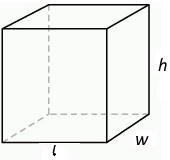#Surface Area of a Rectangular Box CalculatorLength: units Width: units Height: units Area: units2

This application calculates the surface area of a rectangular box, of course a box such as a packing box may be considered to have two surface areas, the outer surface and the inner surface. This application calculates only the outer surface area. If the thickness of the material from which the box is made is zero, then the inside and outside surface areas would be the same.

To find the surface area of a rectangular box, you need to find the area of each of its 6 sides. The formula for calculating the surface area of a rectangular box is:

a = 2lw + 2wh + 2lh

where:
l = length of the box
w = width of the box
h = height of the box
a = area of surface

When entering numbers into the calculator, do not enter the units. The units can be considered any units, as long as they are all the same unit. The result will be the surface area in that same unit squared. Do not enter commas in your numbers.